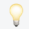This website requires JavaScript.# Search for excited $\tau$-leptons and leptoquarks in the final state with $\tau$-leptons and jets in pp collisions at $\sqrt{s} = 13$ TeV with the ATLAS detector

Mar 2023

A search is reported for excited $\tau$-leptons and leptoquarks in eventswith two hadronically decaying $\tau$-leptons and two or more jets. The searchuses proton-proton (pp) collision data at $\sqrt{s} = 13$ TeV recorded by theATLAS experiment during the Run 2 of the Large Hadron Collider in 2015-2018.The total integrated luminosity is 139 fb$^{-1}$. The excited $\tau$-lepton isassumed to be produced and to decay via a four-fermion contact interaction intoan ordinary $\tau$-lepton and a quark-antiquark pair. The leptoquarks areassumed to be produced in pairs via the strong interaction, and each leptoquarkis assumed to couple to a charm or lighter quark and a $\tau$-lepton. No excessover the background prediction is observed. Excited $\tau$-leptons with massesbelow 2.8 TeV are excluded at 95% CL in scenarios with the contact interactionscale $\Lambda$ set to 10 TeV. At the extreme limit of model validity where$\Lambda$ is set equal to the excited $\tau$-lepton mass, excited$\tau$-leptons with masses below 4.6 TeV are excluded. Leptoquarks with massesbelow 1.3 TeV are excluded at 95% CL if their branching ratio to a charm quarkand a $\tau$-lepton equals 1. The analysis does not exploit flavour-tagging inthe signal region.Q1论文试图解决什么问题？
Q2这是否是一个新的问题？
Q3这篇文章要验证一个什么科学假设？
0